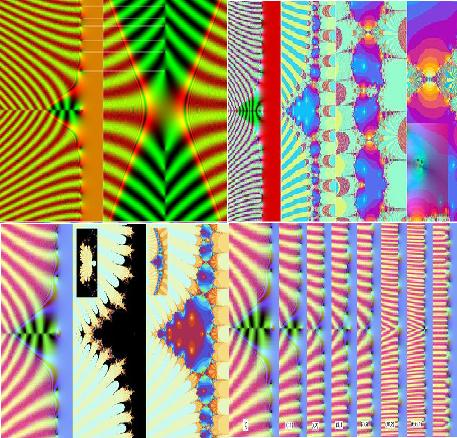# Experimental Observations on the Uncomputability of the Riemann Hypothesis from Administrator's blog

Experimental Observations on the Uncomputability of the Riemann Hypothesis (by Chris King)::

This paper seeks to explore whether the Riemann hypothesis falls into a class of putatively unprovable mathematical conjectures, which arise as a result of unpredictable irregularity. It also seeks to provide an experimental basis to discover some of the mathematical enigmas surrounding these conjectures, by providing Matlab and C programs which the reader can use to explore and better understand these systems (see appendix 6 in Part II).

Part I of this article includes: Introduction; The Riemann hypothesis and the Zeta Function; and The Quantum Chaos Connection.

Part II of this article includes: Julia and Mandelbrot sets of the Riemann Zeta Function; The Syracuse Shibboleth; and Appendices: 1. Moments of Zeta; 2. Zeta Bernoulli Numbers; 3. Twin Prime Distribution; 4. Other Dirichlet series for zeta; 5. Lanczos approximation to Gamma; 6. Mac XCode Viewer and Matlab Toolbox for Figures; and 7. A Formula for Depicting the Derivative of Zeta.

Blog home